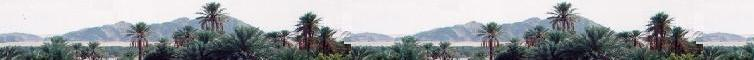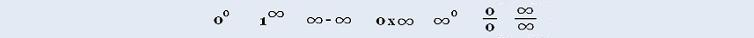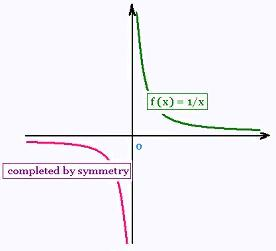###### Calculus I

Exercices

Applications

Marginal analysis

© The scientific sentence. 2010

# Calculus I: Symmetry and graph

### 1. Parity of a function

A function f is even if for all x in its domain, f(- x) = f(x)

A function f is odd if for all x in its domain, f(- x)= - f(x)

Examples:

a) f(x) = x2 + 4 is even because f(- x) = (- x)2 + 4 = x2 + 4

b)f(x) = sin x is odd because sin(- x) = - sin (x)

c) f(x) = 2x + 3 is neither even nor odd.

### 2. SymmetryThe graph of an even function is symmetrical with respect to the axis y while the graph of an odd function is symmetric with respect to the origin.

When a function is odd or even, we study the function in its interval of symmetry and complete by symmetry.

Example: f(x) = 1/x is odd. Its interval os symetry is I = [0, + ∞[. We study and graph this function on I and complete by symmetry with respect to the origin.

### 3. Exercices

 chimie labs | Physics and Measurements | Probability & Statistics | Combinatorics - Probability | Chimie | Optics | contact |